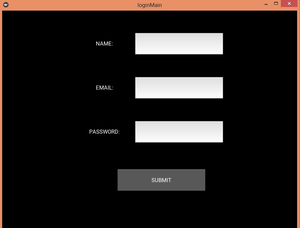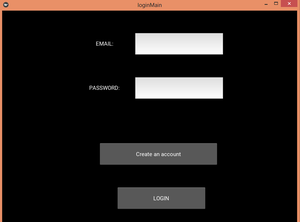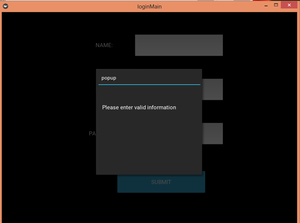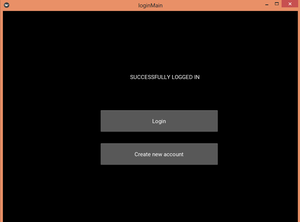Related Articles
Login Application and Validating info using Kivy GUI and Pandas in Python
• Last Updated : 17 Aug, 2020

Prerequisites : Kivy, Pandas

Kivy is a multiplatform GUI library, known for being responsive. It provides management of multiple screens in a single application.

In this application we will be using multiple screens to log in user’s info and validate it. We will save the information in a csv file and use pandas to validate the information inside of the csv file by reading it into a DataFrame. To build the GUI we will use .kv file.

Approach :

1. There will be three screens, one for letting the user log in, second for signing up and third for telling if the login was successful.
2. The info will be stored in a csv file.
3. Pandas Library will be used to read the csv file into a DataFrame and further check if the user info already exists or not.
4. If the information entered is invalid, popups will inform the user.
5. Finally, the user will be informed if the login was successful or not.

Main program :

 `# import all the relevant classes``from` `kivy.app ``import` `App``from` `kivy.uix.widget ``import` `Widget``from` `kivy.uix.screenmanager ``import` `ScreenManager, Screen``from` `kivy.properties ``import` `ObjectProperty``from` `kivy.lang ``import` `Builder``from` `kivy.uix.popup ``import` `Popup``from` `kivy.uix.floatlayout ``import` `FloatLayout``import` `pandas as pd`` ` `# class to call the popup function``class` `PopupWindow(Widget):``    ``def` `btn(``self``):``        ``popFun()`` ` `# class to build GUI for a popup window``class` `P(FloatLayout):``    ``pass`` ` `# function that displays the content``def` `popFun():``    ``show ``=` `P()``    ``window ``=` `Popup(title ``=` `"popup"``, content ``=` `show,``                   ``size_hint ``=` `(``None``, ``None``), size ``=` `(``300``, ``300``))``    ``window.``open``()`` ` `# class to accept user info and validate it``class` `loginWindow(Screen):``    ``email ``=` `ObjectProperty(``None``)``    ``pwd ``=` `ObjectProperty(``None``)``    ``def` `validate(``self``):`` ` `        ``# validating if the email already exists ``        ``if` `self``.email.text ``not` `in` `users[``'Email'``].unique():``            ``popFun()``        ``else``:`` ` `            ``# switching the current screen to display validation result``            ``sm.current ``=` `'logdata'`` ` `            ``# reset TextInput widget``            ``self``.email.text ``=` `""``            ``self``.pwd.text ``=` `""`` ` ` ` `# class to accept sign up info  ``class` `signupWindow(Screen):``    ``name2 ``=` `ObjectProperty(``None``)``    ``email ``=` `ObjectProperty(``None``)``    ``pwd ``=` `ObjectProperty(``None``)``    ``def` `signupbtn(``self``):`` ` `        ``# creating a DataFrame of the info``        ``user ``=` `pd.DataFrame([[``self``.name2.text, ``self``.email.text, ``self``.pwd.text]],``                            ``columns ``=` `[``'Name'``, ``'Email'``, ``'Password'``])``        ``if` `self``.email.text !``=` `"":``            ``if` `self``.email.text ``not` `in` `users[``'Email'``].unique():`` ` `                ``# if email does not exist already then append to the csv file``                ``# change current screen to log in the user now ``                ``user.to_csv(``'login.csv'``, mode ``=` `'a'``, header ``=` `False``, index ``=` `False``)``                ``sm.current ``=` `'login'``                ``self``.name2.text ``=` `""``                ``self``.email.text ``=` `""``                ``self``.pwd.text ``=` `""``        ``else``:``            ``# if values are empty or invalid show pop up``            ``popFun()``     ` `# class to display validation result``class` `logDataWindow(Screen):``    ``pass`` ` `# class for managing screens``class` `windowManager(ScreenManager):``    ``pass`` ` `# kv file``kv ``=` `Builder.load_file(``'login.kv'``)``sm ``=` `windowManager()`` ` `# reading all the data stored``users``=``pd.read_csv(``'login.csv'``)`` ` `# adding screens``sm.add_widget(loginWindow(name``=``'login'``))``sm.add_widget(signupWindow(name``=``'signup'``))``sm.add_widget(logDataWindow(name``=``'logdata'``))`` ` `# class that builds gui``class` `loginMain(App):``    ``def` `build(``self``):``        ``return` `sm`` ` `# driver function``if` `__name__``=``=``"__main__"``:``    ``loginMain().run()`

.kv file : .kv file contains all the code to design and place the GUI and to define the direction of the transitions of the screens.

 `# there are three screens``windowManager:``    ``loginWindow:``    ``signupWindow:``    ``logDataWindow:`` ` `# GUI for the login window``:``    ``email : email``    ``pwd : pwd``    ``FloatLayout:``        ``size: root.width, root.height``        ``Label:``            ``text : ``"EMAIL: "``            ``size_hint : ``0.2``, ``0.1``            ``pos_hint : {``"x"``:``0.25``, ``"top"``:``0.9``}``        ``TextInput:``            ``id` `: email``            ``multiline :``False``            ``size_hint : ``0.3``, ``0.1``            ``pos_hint : {``"x"` `: ``0.45``, ``"top"` `: ``0.9``}``        ``Label:``            ``text : ``"PASSWORD: "``            ``size_hint : ``0.2``, ``0.1``            ``pos_hint : {``"x"` `: ``0.25``, ``"top"` `: ``0.7``}``        ``TextInput:``            ``id` `: pwd``            ``multiline :``False``            ``size_hint : ``0.3``, ``0.1``            ``pos_hint : {``"x"` `: ``0.45``, ``"top"` `: ``0.7``}``        ``Button:``            ``text : ``"Create an account"``            ``size_hint : ``0.4``, ``0.1``            ``pos_hint : {``"x"` `: ``0.33``, ``"top"` `: ``0.4``}``            ``on_release: ``                ``app.root.current ``=` `'signup'``                ``root.manager.transition.direction ``=` `"left"``        ``Button:``            ``text : ``"LOGIN"``            ``size_hint : ``0.3``, ``0.1``            ``pos_hint : {``"x"` `: ``0.39``, ``"top"` `: ``0.2``}``            ``on_release: ``                ``root.validate()``                ``root.manager.transition.direction ``=` `"up"`` ` `# GUI for the signup window``:``    ``name2 : name2``    ``email : email``    ``pwd : pwd``    ``FloatLayout:``        ``Label:``            ``text : ``"NAME: "``            ``size_hint : ``0.2``, ``0.1``            ``pos_hint : {``"x"``:``0.25``, ``"top"``:``0.9``}``        ``TextInput:``            ``id` `: name2``            ``multiline : ``False``            ``size_hint : ``0.3``, ``0.1``            ``pos_hint : {``"x"` `: ``0.45``, ``"top"` `: ``0.9``}``        ``Label:``            ``text : ``"EMAIL: "``            ``size_hint : ``0.2``, ``0.1``            ``pos_hint : {``"x"` `: ``0.25``, ``"top"` `: ``0.7``}``        ``TextInput:``            ``id` `: email``            ``multiline : ``False``            ``size_hint : ``0.3``, ``0.1``            ``pos_hint : {``"x"` `: ``0.45``, ``"top"` `: ``0.7``}``        ``Label:``            ``text : ``"PASSWORD: "``            ``size_hint : ``0.2``, ``0.1``            ``pos_hint : {``"x"` `: ``0.25``, ``"top"` `: ``0.5``}``        ``TextInput:``            ``id` `: pwd``            ``multiline : ``False``            ``size_hint : ``0.3``, ``0.1``            ``pos_hint : {``"x"` `: ``0.45``, ``"top"` `: ``0.5``}``        ``Button:``            ``text : ``"SUBMIT"``            ``size_hint : ``0.3``, ``0.1``            ``pos_hint : {``"x"` `: ``0.39``, ``"top"` `: ``0.28``}``            ``on_press :``                ``root.signupbtn()``                ``root.manager.transition.direction ``=` `"right"`` ` `# GUI to show validation result``:``    ``info : info``    ``FloatLayout:``        ``Label:``            ``id` `: info``            ``size_hint : ``0.8``, ``0.2``            ``pos_hint : {``"x"` `: ``0.15``, ``"top"` `: ``0.8``}``            ``text : ``"SUCCESSFULLY LOGGED IN"``        ``Button:``            ``text : ``"Login"``            ``size_hint : ``0.4``, ``0.1``            ``pos_hint : {``"x"` `: ``0.33``, ``"top"` `: ``0.55``}``            ``on_release: ``                ``app.root.current ``=` `'login'``                ``root.manager.transition.direction ``=` `"down"``        ``Button:``            ``text : ``"Create new account"``            ``size_hint : ``0.4``, ``0.1``            ``pos_hint : {``"x"` `: ``0.33``, ``"top"` `: ``0.4``}``            ``on_release: ``                ``app.root.current ``=` `'signup'``                ``root.manager.transition.direction ``=` `"down"`` ` ` ` `# GUI for pop up window``

:``    ``Label:``        ``text : ``"Please enter valid information"``        ``size_hint : ``0.2``, ``0.1``        ``pos_hint : {``"x"` `: ``0.3``, ``"top"` `: ``0.8``}`

Output:

Signup Window :Login Window :Popup Window:Validation :Attention geek! Strengthen your foundations with the Python Programming Foundation Course and learn the basics.

To begin with, your interview preparations Enhance your Data Structures concepts with the Python DS Course.

My Personal Notes arrow_drop_up